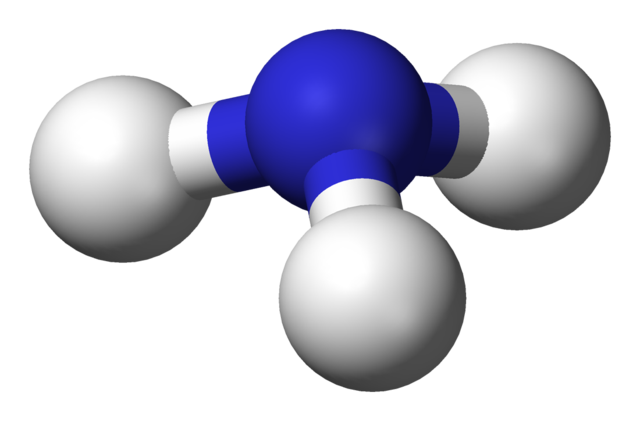Chemistry AQA GCSE:
Haber Process + Fertilisers

Page 1

Q1-11: Ammonia is produced industrially in the Haber Process. The reaction is exothermic.

N2(g)   +   3H2(g)2NH3(g)1. What is the main source of the hydrogen used in the Haber Process?
• A.   natural gas
• B.   water
• C.   air
• D.   hydrochloric acid
2. What is the main source of the nitrogen used in the Haber Process?
• A.   fertilizers
• B.   natural gas
• C.   air
• D.   coal
3. What happens to the unreacted nitrogen and hydrogen gases in the Haber Process?
• A.  they are collected and sold
• B.   they are recycled back into the reactor
• C.   they are collected and used at a later date
• D.    they are thrown away as waste products
4. Approximately what temperature in degrees celcius is used in the Haber Process?
5. Why is a higher temperature NOT used in the Haber Process?
• A.   The equilibrium would move to the left reducing the amount of ammonia
• B.   The equilibrium would move to the right increasing the amount of ammonia
• C.   The rate of reaction would be slower at a higher temperature.
• D.   The rate of reaction would be faster at a higher temperature.
6. Why is a lower temperature NOT used in the Haber Process?
• A.   The equilibrium would move to the left reducing the amount of ammonia
• B.   The equilibrium would move to the right increasing the amount of ammonia
• C.   The rate of reaction would be too slow at a lower temperature.
• D.   The rate of reaction would be too fast at a lower temperature.
7. Approximately what pressure is used in the Haber Process?

8. What would happen to the rate at which equilibrium is reached, and the position of equilibrium, if a lower pressure was used?

 Rate Position of equilibrium A increases Moves to the right B increases Moves to the left C decreases Moves to the right D decreases Moves to the left
9. Why is a higher pressure NOT used in the Haber Process?
• A.   The equilibrium would move to the right increasing the amount of ammonia
• B.   The equilibrium would move to the left decreasing the amount of ammonia
• C.   High pressures are expensive to maintain
• D.   The rate of reaction would increase
10. A catalyst is used in the Haber Process. What material is used for the catalyst?
• A.   iron
• B.   nickel
• C.   copper
• D.   manganese oxide

11. What effect does the catalyst have on the rate of reaction and the position of equilibrium?

 Rate Position of equilibrium A increases Moves to the right B increases No effect C decreases Moves to the right D decreases No effect